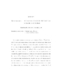## Autonomous Stochastic Perturbations of Hamiltonian Systems

 dc.contributor.advisor Freidlin, Mark I en_US dc.contributor.author Ren, Huaizhong en_US dc.contributor.department Mathematics en_US dc.contributor.publisher Digital Repository at the University of Maryland en_US dc.contributor.publisher University of Maryland (College Park, Md.) en_US dc.date.accessioned 2004-08-27T05:17:48Z dc.date.available 2004-08-27T05:17:48Z dc.date.issued 2004-06-29 en_US dc.description.abstract We consider autonomous stochastic perturbations $\dot X^{\varepsilon}(t)=\skewgrad H(X^{\varepsilon}(t))+\varepsilon b(X^{\varepsilon}(t))$ of Hamiltonian systems of one degree of freedom whose Hamiltonian $H$ is quadratic in a neighborhood of the only saddle point of $H$. Assume that $b=b_1+\xi b_2$ for some random fields $b_i$, $i=1,\,2$, and $\xi$ is a random variable, and that ${\rm div}b_i<0$ and $\xi>0$ with probability $1$. Also assume that $H$ has only one saddle point and two minima. To consider the effects of the perturbations, we consider the graph $\Gamma$ homeomorphic to the space of connected components of the level curves of $H$ and the processes $Y^{\varepsilon}_t$ on $\Gamma$ which represent the slow component of the motion of the perturbed system. We show that as $\varepsilon\rightarrow 0$, the processes $Y^{\varepsilon}_t$ tend to a certain stochastic process $Y_t$ on $\Gamma$ which can be determined inside the edges by a version of the averaging principle and branches at the interior vertex into adjacent edges with certain probabilities which can be calculated by $H$ and the perturbation $b$. Also our result can be used to regularize some deterministic perturbations, partially coinciding with the results obtained by Brin and Freidlin in a earlier work. en_US dc.format.extent 602034 bytes dc.format.mimetype application/pdf dc.identifier.uri http://hdl.handle.net/1903/1708 dc.language.iso en_US dc.subject.pqcontrolled Mathematics en_US dc.subject.pqcontrolled Mathematics en_US dc.subject.pquncontrolled autonomous stochastic perturbations en_US dc.subject.pquncontrolled Hamiltonian systems en_US dc.subject.pquncontrolled averaging principle en_US dc.subject.pquncontrolled processes on a graph en_US dc.subject.pquncontrolled branching probabilities en_US dc.subject.pquncontrolled weak convergence en_US dc.title Autonomous Stochastic Perturbations of Hamiltonian Systems en_US dc.type Dissertation en_US
##### Original bundle
Now showing 1 - 1 of 1